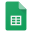For each nonconformance (nc) we have collected two measures about the complexity to discover the nonconformance: breadth (B) and depth (D). The first is related to the number of calls into a test method realized until the nonconformance exposure. This metric is defined in Equation 1; the calls(tm) returns the sequence of method calls into the test method received as parameter. The second, is related to the depth required to find each nonconformance the internal calls performed until the contract have been violated. This metric is defined in Equation 2. Given the method that corresponds to the position in which the nonconformance was revealed, if the nonconformance is into the body of this method D receives 1, otherwise, its value is recursively increased until the method that reveals the nonconformance.

In Table 1 we present the values for metrics B and D collected for all 84 nonconformances detected by JMLOK tool in samples and real Java/JML programs.

Table 1. Nonconformances on Samples and Real programs. Column Experimental Unit shows the name of each experimental unit. Column Class shows class name that has one (or more) nonconformance(s). Column Method show the method name that has one (or more) nonconformance(s). Column Breadth (B) shows the value of the Breadth metric. And finally column Depth (D) shows the value of D metric.Metrics-B-D-Nonconformances

We calculate the average for these metrics and obtained the following results:Metrics Summary